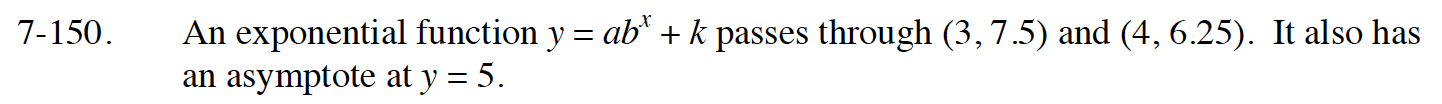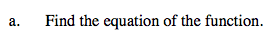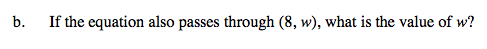Home > CCA2 > Chapter 7 > Lesson 7.2.3 > Problem7-150

7-150.
1. An exponential function y = abx + k passes through (3, 7.5) and (4, 6.25). It also has an asymptote at y = 5. Homework Help ✎

1. Find the equation of the function.

2. If the equation also passes through (8, w), what is the value of w?Substitute 5 for k and then use the points to write a system of equations.

Rewrite each equation by subtracting 5 from both sides.

Solve the first equation for a.

Substitute for a in the second equation.

Solve for b.

$\text{Substitute }\frac{1}{2}\text{ for }b \text{ to solve for }a.$

$y = 20\left(\frac{1}{2}\right)^x + 5$

k = 5
7.5 = ab3 + 5
6.25 = ab4 + 5

ab3 = 2.5
ab4 = 1.25

$a\ = \frac{2.5}{b^3}$

$\left( \frac{2.5}{b^3} \right)b^4\ =\ 1.25$

$b\ = \frac{1}{2}$

$a\ = \frac{2.5}{\left(\frac{1}{2}\right)^3}$

a = 20Substitute 8 for x and w for y.

$w = 20\left(\frac{1}{2}\right)^8 + 5$